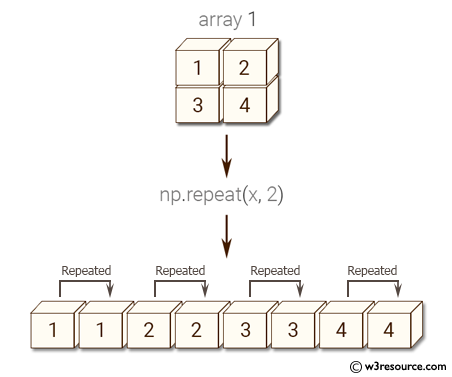﻿ NumPy: Repeat elements of an array - w3resource# NumPy: Repeat elements of an array

## NumPy: Array Object Exercise-26 with Solution

Write a NumPy program to repeat elements of an array.

Pictorial Presentation:Sample Solution:-

Python Code:

``````import numpy as np
x = np.repeat(3, 4)
print(x)
x = np.array([[1,2],[3,4]])
print(np.repeat(x, 2))
```
```

Sample Output:

```[3 3 3 3]
[1 1 2 2 3 3 4 4]
```

Python Code Editor:

Have another way to solve this solution? Contribute your code (and comments) through Disqus.

What is the difficulty level of this exercise?

Test your Python skills with w3resource's quiz

﻿

## Python: Tips of the Day

List comprehension:

```>>> m = [x ** 2 for x in range(5)]
>>> m
[0, 1, 4, 9, 16]
```